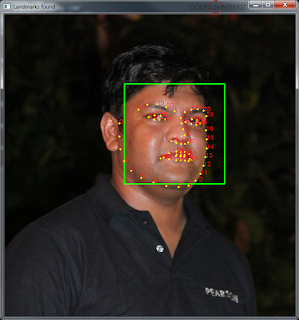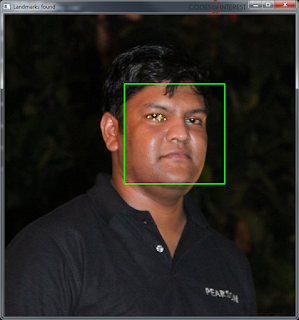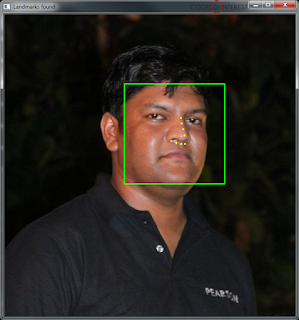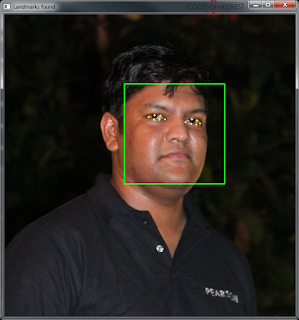# Extracting individual Facial Features from Dlib Face Landmarks

If you remember, in my last post on Dlib, I showed how to get the Face Landmark Detection feature of Dlib working with OpenCV. We saw how to use the pre-trained 68 facial landmark model that comes with Dlib with the shape predictor functionality of Dlib, and then to convert the output of into a numpy array to use it in an OpenCV context. We were able to get all 68 feature points on to our face image.Dlib detecting the 68 Face Landmarks

The 68 feature points which the Dlib model detects include the Jawline of the face, left and right eyes, left and right eyebrows, the nose, and the mouth. So, what if you only want to detect few of those features on a face? E.g. you may only want to detect the positions of the eyes and the nose. Is there a way to extract only few of the features from the Dlib shape predictor?

There is actually a very simple way to do that. Here’s how.

Let’s take the same image above, and add a bit of code to annotate the 68 feature points.
Update 5/Apr/17: The code I posted initially gave some errors when trying to run on OpenCV 3 and Python 3+. Thanks Shirish Ranade for pointing it out and sharing the code fix. The code is now updated so that it works on Python 2.7 and 3+ with either OpenCV 2 or 3.

`````` faceCascade = cv2.CascadeClassifier(cascPath)

predictor = dlib.shape_predictor(PREDICTOR_PATH)

gray = cv2.cvtColor(image, cv2.COLOR_BGR2GRAY)

# Detect faces in the image
gray,
scaleFactor=1.05,
minNeighbors=5,
minSize=(100, 100),
)

print("Found {0} faces!".format(len(faces)))

# Draw a rectangle around the faces
for (x, y, w, h) in faces:
cv2.rectangle(image, (x, y), (x + w, y + h), (0, 255, 0), 2)

# Converting the OpenCV rectangle coordinates to Dlib rectangle
dlib_rect = dlib.rectangle(int(x), int(y), int(x + w), int(y + h))

landmarks = np.matrix([[p.x, p.y]
for p in predictor(image, dlib_rect).parts()])

for idx, point in enumerate(landmarks):
pos = (point[0, 0], point[0, 1])
cv2.putText(image, str(idx), pos,
fontFace=cv2.FONT_HERSHEY_SCRIPT_SIMPLEX,
fontScale=0.4,
color=(0, 0, 255))

cv2.circle(image, pos, 2, color=(0, 255, 255), thickness=-1)

cv2.imshow("Landmarks found", im)
cv2.waitKey(0)
``````

We are simply numbering each of the feature points, in the order they come from the shape predictor, from '0' to '67'. The result will look like this.The 68 feature points, annotated
If you look closely at the numbers (and check with several images), you’ll notice that the feature points are always coming in the same order. That is,
• Points 0 to 16 is the Jawline
• Points 17 to 21 is the Right Eyebrow
• Points 22 to 26 is the Left Eyebrow
• Points 27 to 35 is the Nose
• Points 36 to 41 is the Right Eye
• Points 42 to 47 is the Left Eye
• Points 48 to 60 is Outline of the Mouth
• Points 61 to 67 is the Inner line of the Mouth
And these always come in that order.

So, all we need to do is to slice the array that contains the feature points in order to get the points of the features we want.

Let’s first define the ranges of each of those features,
`````` JAWLINE_POINTS = list(range(0, 17))
RIGHT_EYEBROW_POINTS = list(range(17, 22))
LEFT_EYEBROW_POINTS = list(range(22, 27))
NOSE_POINTS = list(range(27, 36))
RIGHT_EYE_POINTS = list(range(36, 42))
LEFT_EYE_POINTS = list(range(42, 48))
MOUTH_OUTLINE_POINTS = list(range(48, 61))
MOUTH_INNER_POINTS = list(range(61, 68))
``````

Now, all we need to do is to slice the array using these ranges.

Let’s say we only need to get the right eye,
`````` landmarks = np.matrix([[p.x, p.y]
for p in predictor(image, dlib_rect).parts()])

landmarks_display = landmarks[RIGHT_EYE_POINTS]

for idx, point in enumerate(landmarks_display):
pos = (point[0, 0], point[0, 1])
cv2.circle(image, pos, 2, color=(0, 255, 255), thickness=-1)
``````Getting just the Right Eye
Just the nose? Easy, just change,
`````` landmarks_display = landmarks[NOSE_POINTS]
``````Just the Nose
But, what if you want to get a combination of features? Let's say, you want to get both the eyes.

That's also quite simple, you just add it up,
`````` landmarks_display = landmarks[RIGHT_EYE_POINTS + LEFT_EYE_POINTS]
``````Getting both Left and Right Eyes
Let's try the Nose and the Outline of the Mouth,
`````` landmarks_display = landmarks[NOSE_POINTS + MOUTH_OUTLINE_POINTS]
``````

Quite simple right?

You can use this same technique to extract any combination of face feature points from the Dlib Face Landmark Detection.

Here's the full source code to get you started,
`````` import numpy as np
import cv2
import dlib

imagePath = "path to your image"

PREDICTOR_PATH = "path to your shape_predictor_68_face_landmarks.dat file"

JAWLINE_POINTS = list(range(0, 17))
RIGHT_EYEBROW_POINTS = list(range(17, 22))
LEFT_EYEBROW_POINTS = list(range(22, 27))
NOSE_POINTS = list(range(27, 36))
RIGHT_EYE_POINTS = list(range(36, 42))
LEFT_EYE_POINTS = list(range(42, 48))
MOUTH_OUTLINE_POINTS = list(range(48, 61))
MOUTH_INNER_POINTS = list(range(61, 68))

predictor = dlib.shape_predictor(PREDICTOR_PATH)

gray = cv2.cvtColor(image, cv2.COLOR_BGR2GRAY)

# Detect faces in the image
gray,
scaleFactor=1.05,
minNeighbors=5,
minSize=(100, 100),
)

print("Found {0} faces!".format(len(faces)))

# Draw a rectangle around the faces
for (x, y, w, h) in faces:
cv2.rectangle(image, (x, y), (x + w, y + h), (0, 255, 0), 2)

# Converting the OpenCV rectangle coordinates to Dlib rectangle
dlib_rect = dlib.rectangle(int(x), int(y), int(x + w), int(y + h))

landmarks = np.matrix([[p.x, p.y]
for p in predictor(image, dlib_rect).parts()])

landmarks_display = landmarks[RIGHT_EYE_POINTS + LEFT_EYE_POINTS]

for idx, point in enumerate(landmarks_display):
pos = (point[0, 0], point[0, 1])
cv2.circle(image, pos, 2, color=(0, 255, 255), thickness=-1)

cv2.imshow("Landmarks found", image)
cv2.waitKey(0)
``````

Related posts:
Getting Dlib Face Landmark Detection working with OpenCV

http://www.pyimagesearch.com/2017/04/03/facial-landmarks-dlib-opencv-python/
https://matthewearl.github.io/2015/07/28/switching-eds-with-python/

Build Deeper: Deep Learning Beginners' Guide is the ultimate guide for anyone taking their first step into Deep Learning.

1.hi if i have Cascades file of traffic sign , is it possible to detect with traffic sign same as facial landmark??

1.If you have a Cascade file for traffic signs, you would be able to use it to detect traffic signs and draw the bounding box of the traffic sign.
But to detect specific points within the traffic sign (a.k.a. landmarks) you would need a shape predictor specifically trained for traffic signs. The shape_predictor_68_face_landmarks unfortunately only works for faces.

2.hi sir if i have dlib library using how to get the human face detect after how to generate dataset.

3.Okay .....is it possible to detect and recognize the traffic signs with dlib??

4.HI, your work is good. I have a question. Can I connect point with each other with lines. It is possible???

5.where can i get the xml filefrom